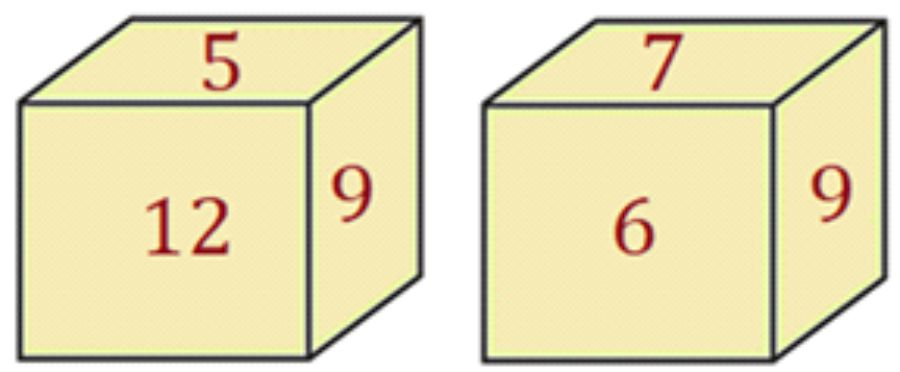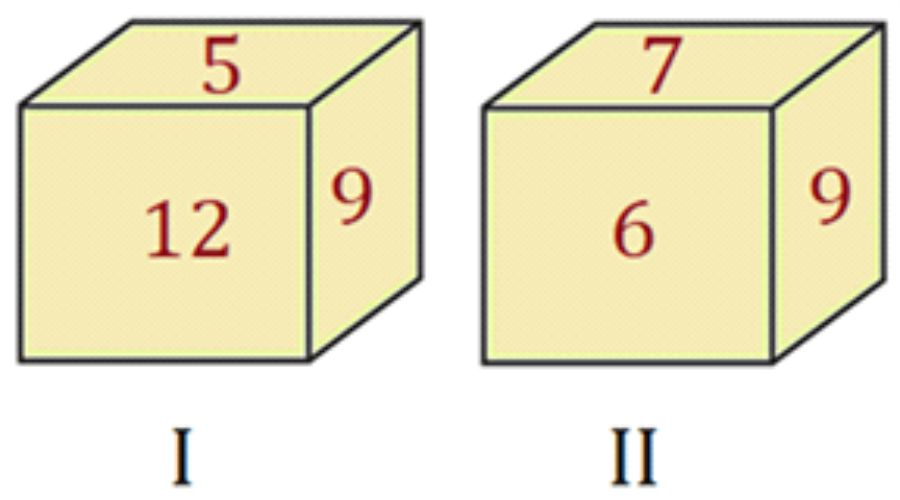We do not support landscape mode yet.
Please go back to portrait mode for the best experience
Question of The Day09-12-2019

Two positions of the same dice are shown below. There are different numbers printed on each face of the dice. If a number printed on one of the faces is ‘1’, then what will be the least sum of digits on opposite faces?Correct Answer : b ) 10

Explanation :

Consider the given positions of diceTo find out the numbers opposite to faces on the dice, we should follow the following steps:

• First, find the common number/symbol on both the positions of dice
• Then, starting from the common number move in the clockwise direction in both the dice
• While moving in clockwise direction note down the other numbers/symbols
• Lastly, compare the numbers/symbols thus obtained. The next numbers after the common number in both the positions of the dice will be on the opposite faces of the dice.

NOTE: The above logic cannot be applied on the positions of the dice on which more than one face has common number or symbols. For such cases the non-common numbers will be opposite to each other.

Therefore, analyzing position I and position II of dice we can say that there is ‘9’ common in both the positions of dice

So,

For position I:

The sequence of the numbers by the above logic will be

9, 12, 5

For position II:

The sequence of the numbers by the above logic will be

9, 6, 7

Now, from the above logic, we can say that

12 is opposite to 6

5 is opposite to 7

According to the question as there is ‘1’ printed on one face of the dice so,

1 is opposite to 9

Therefore, sum of the digits on opposite faces will be

9 + 1 ⇒ 10

12 + 6 ⇒ 18

5 + 7 ⇒ 12

Thus, the least sum will be ⇒ 10

Hence, (b) is the correct answer.

Such type of question is asked in SSC CGL, SSC MTS, SSC CPO, SSC CHSL, RRB JE, RRB NTPC, RRB GROUP D, etc.  Try and attempt free mock tests at PendulumEdu and read Daily Current Affairs and Banking Affairs to boost your exam preparation.